# The Inversion of Functions Defined by Turing Machines

J. McCarthy
Computer Science Department
Stanford University
Stanford, CA 95305
jmc@cs.stanford.edu
http://www-formal.stanford.edu/jmc/

1956

Consider the problem of designing a machine to solve well-defined intellectual problems. We call a problem well-defined if there is a test which can be applied to a proposed solution. In case the proposed solution is a solution, the test must confirm this in a finite number of steps. If the proposed solution is not correct, we may either require that the test indicate this in a finite number of steps or else allow it to go on indefinitely. Since any test may be regarded as being performed by a Turing machine, this means that well-defined intellectual problems may be regarded as those of inverting functions and partial functions defined by Turing machines.

Let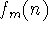be the partial function computed by the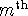Turing machine. It is not defined for a given value of n if the computation does not come to an end. This paper deals with the problem of designing a Turing machine which, when confronted by the number pair (m, r), computes as efficiently as possible a function g(m, r) such that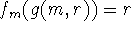. Again, for particular values of m and r no g(m, r) need exist. In fact, it has been shown that the existence of g(m, r) is an undecidable question in that there does not exist a Turing machine which will eventually come to a stop and print a 1 if g(m, r) does not exist.

In spite of this, it is easy to show that a Turing machine exists which will compute a g(m, r) if such exists. Essentially, it substitutes integers inuntil it comes to one such that fm(n) = r. It will therefore find g(m, r) if it exists, but will never know enough to give up if g(m, r) does not exist. Since the computation ofmay not terminate for some n, it is necessary to avoid getting stuck on such n's Hence the machine calculates the numbers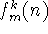in some order whereisif the computation ofends after k steps and is otherwise undefined

Our problem does not end once we have found this procedure for computing g(m, r) because this procedure is extremely inefficient. It corresponds to looking for a proof of a conjecture by checking in some order all possible English essays

In order to do better than such a purely enumerative method, it is necessary to use methods which take into account some of the structure of Turing machines. But before discussing this, we must attempt to be somewhat (only somewhat) more precise about what is meant by efficiency

The most obvious idea is to say that if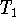and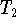are two Turing machines each computing a g(m, r), then for a particular m and r the more efficient one is the one which carries out the computation in the fewest steps. However, this won't do since for any Turing machine there is another one which does k steps of the original machine in one step However, the new machine has many more different kinds of symbol than the old. It is probably also possible to increase the speed by increasing the number of internal states, though this is not so easy to show. (Shannon shows elsewhere in these studies that it is possible to reduce the number of internal states to two at the cost of increasing the number of symbols and reducing the speed.)

Hence we offer the following revised definition of the length of a computation performed by a Turing machine. For any universal Turing machine there is a standard way of recoding on it the computation performed by the given machine. Let this computation be recoded on a fixed universal Turing machine and count the number of steps in the new computation. There are certain difficulties here connected with the fact that the rate of computation is limited if the tape of the universal machine is finite dimensional, and hence the rate should probably be defined with respect to a machine whose tape is infinite dimensional but each square of which has at most two states and which has only two internal states. This requires a mild generalization of the concept of Turing machines

The tape space is not required to be either homogeneous or isotropic We hope to make these considerations precise in a later paper. For now, we only remark that dimensionality is meant to bewhere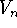is the number of squares accessible to a given one in n steps. It will be made at least plausible that a machine with Q internal states and S symbols should be considered as making about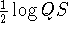elementary steps per step of computation and hence the number of steps in a computation should be multiplied by this factor to get the length of the computation.

Having now an idea of what should be meant by the length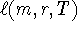of a particular computation of g(m, r) by the machine T, we can return to the question of comparing two Turing machines. Of course, if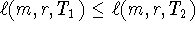for all m and r for which these numbers are finite, we should certainly say thatis more efficient than. (We only consider machines that actually compute g(m, r) whenever it exists. Any machine can be modified into such a machine by adding to it facilities for testing a conclusion and having it spend a small fraction of its time trying the integers in order.) However, it is not so easy to give a function which gives an over-all estimate of the efficiency of a machine at computing g(m, r). The idea of assigning a weight function p(m, r) and then calculating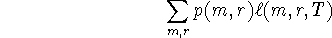does not work very well becauseis not bounded by any recursive function of m and r. (Otherwise, a machine could be described for determining whether the computation ofterminates. It would simply carry out some k(m, n) steps and conclude that if the computation had not terminated by this time it was not going to.) There cannot be any machine which is as fast as any other machine on any problem because there are rather simple machines whose only procedure is to guess a constant which are fast when g(m, r) happens to equal that constant.

We now return to the question of how to design machines which make use of information concerning the structure of Turing machines. The straightforward approach would be to try to develop a formalized theory of Turing machines in which length of a computation is defined and then try to get a decision procedure for this formal theory. This is known to be a hopeless task. Systems much simpler than Turing machine theory have been shown to have unsolvable decision procedures. So, we look for a way of evading these difficulties

Before discussing how this may be done, it is worthwhile to bring up some more enumerative procedures. First, let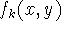be the function of two variables computed by theTuring machine. Our procedure consists in trying the numbers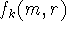in order (again diagonalizing to avoid computations which don't end.) This is based on the plausible idea that, in searching for the solution to a problem, the given data should be taken into account.

The next complication which suggests itself is to revise the order in which recursive functions are considered. One way is to consider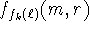diagonalized on k and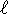. This is based on the idea that the best procedure is more likely to be recursively simple, rather than merely to have a low number in the ordering. More generally, the enumeration of partial recursive functions should give an early place to compositions of the functions which have already appeared.

This process of elaborating the schemes by which numbers are tested can be carried much further. Intuitively it seems that each successive complication improves the performance on difficult problems, but imposes a delay in getting started

The difficulty with the afore-mentioned methods and their elaborations is that they have no concept of partial success. It is a great advantage in conducting a search to be able to know when one is close. This suggests first of all that a functionbe tried out first on simpler problems known to have simple solutions before very many steps of the computationare carried out.

At this point it becomes unclear how to proceed further. What has been done is only a semiformal description of a few of the processes common to scientific reasoning and we have no guarantee of being exhaustive. Of course, exhaustiveness in examining for usefulness can be attained for any effectively enumerable class of objects simply by going through the enumeration. However, enumerative methods are always inefficient. What is needed is a general procedure which ensures that all relevant concepts which can be computed with are examined and that the irrelevant are eliminated as rapidly as possible. Here we remark that a property is useful mainly when it permits a new enumeration of the objects possessing it and not merely a test which can be applied. With the enumeration the objects not possessing the property need not even be examined Of course, it is then necessary to be able to express all other relevant properties as functions of the new enumeration.

In order to get around the fact that all formal systems which are anywhere near adequate for describing recursive function theory are incomplete, we avoid restriction to any one of them by introducing the notion of a formal theory (not for the first time, of course).

For our purposes a formal theory is a decidable predicate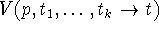which is to be regarded as meaning that p is a proof that the statements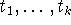imply the statement t in the theory We do not require that statements have negatives or disjunctions, although theories with these and other properties of propositional calculi will presumably belong to the most useful theories.

An interpretation of a formal theory is a computable function i(t) mapping a class of statements of the theory into statements of other theories or concrete propositions. The only kind of concrete proposition which we shall mention for now is P(m, n, r, k) meaning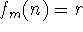in a computation of length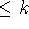. Such a proposition is of course verifiable

The theories and interpretations of them can be enumerated. A function of their Gödel numbers is called a status function. (To be regarded as a current estimate of their validity and relevance, etc.) An action scheme is a computation rule which computes from a status function (its Gödel number) a new status function, perhaps gives an estimate of g(m, r) if it has determined this, and computes a new action scheme.

REMARK 1. A status function would consist primarily of estimates of the validity of the separate theories and would place theories which had not been examined in an ``unknown" category. However, an action scheme might modify the status of a whole class of theories simultaneously in some systematic way.

REMARK 2. The reader should note that a formal equivalence could probably be established between formal theories and certain action schemes Thus, knowledge can be interpreted as a predisposition to act in certain ways under given conditions, and an action scheme can be interpreted as a belief that certain action is appropriate under these conditions. This equivalence should not be made because a simple theory may have a very complicated interpretation as an action scheme and conversely, and in this inversion problem the simplicity of an object is one of its most important properties

This suggests an Alexandrian solution to a knotty question. Perhaps, a machine should be regarded as thinking if and only if its behavior can most concisely be described by statements, including those of the form ``It now believes Pythagoras' theorem." This would not be the case for a phonograph record of a proof of Pythagoras1 theorem. Such statements will probably be especially applicable to predictions of the future behavior under a variety of stimuli.

REMARK 3. In determining a procedure which has action schemes, theories, etc., we are imposing our ideas of the structure of the problem on the machine. The point is to do this so that the procedures we offer are general (will eventually solve every solvable problem) and also are improvable by the methods built into the machine.

REMARK 4. Except for the concrete proposition none of the statements of a formal theory have necessary interpretations which are stateable in advance. However, if the machine were working well, an observer of its internal mechanism might come to the conclusion that a certain statement of a theory is being used as though it were, say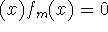, etc. An important merit of a particular theory might be that in it the verification of a proof at the correlate of a certain concrete proposition is much shorter than the computation of the concrete proposition, i.e., shorter than k in P(m, n, r, k).

REMARK 5. The relation of certain action schemes to certain theories might warrant our regarding certain statements in them as being normative, i.e., of the form ``the next axiom scheme should be no. m" or ``theory k should be dropped from consideration."

REMARK 6. Not every worthwhile problem is well-defined in the sense of this paper. In particular, if there exist more or less satisfactory answers with no way of deciding whether an answer already obtained can be improved on a reasonable time, the problem is not well-defined.